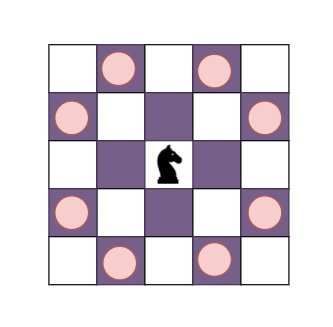# Program to find out the minimum number of moves for a chess piece to reach every position in Python

Suppose, we have a chessboard and a special knight piece K, that moves in an L shape within the board. If the piece is in position (x1, y1) and if it moves to (x2, y2) the movement can be described as x2 = x1 ± a ; y2 = y1 ± b or x2 = x1 ± b ; y2 = y1 ± a ; where a and b are integer numbers. We have to find out the minimum number of moves for that chess piece to reach to reach position (n-1, n-1) on the chessboard from the position (0, 0). If a position is not reachable, it is marked -1, otherwise, the number of moves is returned. We will print n – 1 lines of output, where in each line i it will contain n − 1 integers that describe the minimum number of moves the piece must make for each j.

So, if the input is like n = 6, then the output will be5  4  3  2  5
4 -1  2 -1 -1
3  2 -1 -1 -1
2 -1 -1 -1 -1
5 -1 -1 -1  1

Possible moves for the knight if it is in position (3, 3) in a 5x5 chessboard.

The first line of output contains the minimum number of moves needed by the piece to reach position (1,1) to (1,5). The consecutive lines similarly describe the minimum number of moves for each respective I and j values.

To solve this, we will follow these steps −

• Define a function path_search() . This will take i, j, n

• temp_list := a new map initialized with pairs (-1, -1)

• queue_positional := a new list initialized by pairs (0, 0)

• ver := [(i, j) ,(-i, j) ,(i, -j) ,(-i, -j) ,(j, i) ,(j, -i) ,(-j, i) ,(-j, -i) ]

• while size of queue_positional > 0, do

• current_element := delete first element from queue_positional

• for each element in ver, do

• x := element + current_element

• y := element + current_element

• if -1 < x < n and -1 < y < n and temp_list[x, y] is same as(-1, -1) , then

• temp_list[x, y] := current_element

• insert pair (x, y) at the end of queue_positional

• if x is same as n - 1 and y is same as n - 1, then

• count_var := 1

• while temp_list[x,y] is not same as(0, 0) , do

• count_var := count_var + 1

• x, y := temp_list[x,y]

• return count_var

• return -1

From the main function/method, do the following −

• board := a new map initialized with -1

• for i in range 1 to n, do

• for j in range 1 to i, do

• if board[i, j] is same as -1, then

• board[i, j] := path_search(i, j, n)

• board[j, i] := board[i, j]

• if (n - 1) mod i is same as 0, then

• board[i, i] :=(n - 1) / i

• for i in range 1 to n, do

• for j in range 1 to n - 1, do

• print(board[i, j])

• print(board[i, n - 1])

## Source Code (Python)

Let us see the following implementation to get better understanding −

Live Demo

from collections import defaultdict
def path_search(i, j, n):
temp_list = defaultdict(lambda: (-1,-1))
queue_positional = [(0, 0)]
ver = [(i, j), (-i, j), (i, -j), (-i, -j), (j, i), (j, -i), (-j, i), (-j, -i)]
while len(queue_positional) > 0:
current_element = queue_positional.pop(0)
for element in ver:
x = element + current_element
y = element + current_element
if -1 < x < n and -1 < y < n and temp_list[x, y] == (-1,-1):
temp_list[x, y] = current_element
queue_positional.append((x, y))
if x == n - 1 and y == n - 1:
count_var = 1
while temp_list[x,y]!=(0,0):
count_var += 1
x, y = temp_list[x,y]
return count_var
return -1

def solve(n):
board = defaultdict(lambda: -1)
for i in range(1, n):
for j in range(1, i):
if board[i, j] == -1:
board[i, j] = path_search(i, j, n)
board[j, i] = board[i, j]
if (n - 1) % i == 0:
board[i, i] = (n - 1) / i

for i in range(1, n):
for j in range(1, n - 1):
print(int(board[i, j]), end = ' ')
print(int(board[i, n - 1]))

solve(6)

## Input

6

## Output

5  4  3  2  5
4 -1  2 -1 -1
3  2 -1 -1 -1
2 -1 -1 -1 -1
5 -1 -1 -1  1